# The example for TMatrixDRow TMatrixDColumn TMatrixDDiag TMatrixDSub to get the matrix elements

Hi everyone,

I’d like to have an exmple for the usage of methods I stated in the title. I couldn`t find an example on the website where it was defined.

What I try to do is to define an array and a matrix, then to fill this matrix, then to read all its elements. After that, I will try to work out the calculations with matrices in ROOT.

As a start:

#include “TArrayD.h”
#include “TMatrixD.h”
#include “TMatrixDBase.h”

TMatrixD mat(3,3); // 3X3 matix
TArrayD data(9); // I create an array to store the matrix elements
//BTW, can I se tthe array elements like this: TArrayD data={ 0,1,2,3,4,5,6,7,8}

for (Int_t i = 0; i < data.size(); i++){

data[i] = i;
mat.SetMatrixArray(data.GetArray());
cout << ??? << endl;
}

I`d like to use the structure in that table and on that link.

Cheers.

There is examples here.
May be @moneta can give more of them.

Thanks. Let me check these. I want basic examples for teaching purpose as well. Using pen and paper is easy bit, but it takes time to use the commands and familiarize myself to ROOT for matrix handling .

Even couple of lines showing the application of defining and calling the method will be enough.

NOTE: I have studied the website’s matrix part and linear algebra part. Link.
General question: If not specified explicitly, the matrix elements are constructed and kept in the memory in a row like arrays, aren’t they?

For instance, 3x3 matrix can be visualize  with indexes starting from 0 in group of threes in deed. It’s like ––.

/////////////////////////////////// I have a line of code to practice matrix //////////////////////////////////
//There was a total function defined as 2 Gaussians and one pol2 in advance. 9 parameters in total.
TFitResultPtr r_total = h1->Fit(total, “LRS”);
TMatrixD c_total = r_total->GetCovarianceMatrix();
TMatrixD c_g1 = c_total.GetSub(0, 2, 0, 2); // gaus(0)
TMatrixD c_g2 = c_total.GetSub(3, 5, 3, 5); // gaus(3)
TMatrixD c_bg = c_total.GetSub(6, 8, 6, 8); // pol2(6)
Which part of the 3x3 matrix, these GetSubs are getting when we think the matrix picture as below.

In this example above, there are 9 parameters coming from 3 functions to constitute the covariance matrix. In that 3x3 matrix, diagonal gives the variances and the rest give us the co-variances in a correlation of the two.
When I look at the part of GetSub(0, 2, 0, 2), GetSub(3, 5, 3, 5), GetSub(6, 8, 6, 8), I can see why the row and column lower and upper bounds are 0-2, 3-5, 6-8. It’s the same reason I said about how I visualize the matrix in a row fashion. In that perspective, the row and column lower and upper bounds should repeat themselves because in row fashion structure, the number of row and column is the same (––). In that order, if you are the element at 4th in that row, you are also the 4th column (for instance ).

An example for a cov-matrix in excel I did:

Thus, I can see that it’s 3x3 matrix.

Correct me if I think wrongly.

As you know, we can define the matrix for instance, A=[a_ij]mxn. In math, these i and j sub-indexes start from 1, but in coding, I think, we start them from 0 when we call them. Am I right? (At that point, I don’t know how to use TMatrixDRow(A,i)(j)…)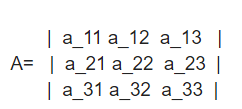const int n = 3;
double a[] = {12, -51, 4, 6, 167, -68, -4, 24, -41};
TMatrixT A(3, 3, a);
std::cout << "matrix A " << std::endl;
A.Print();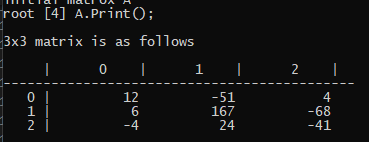//Then, how to get the elements, rows or columns with TMatrixDRow(A,i)(j)…
TMatrixD row1= A.GetSub(0,2,0,2);
TMatrixD row2= A.GetSub(3,5,3,5);
TMatrixD row3= A.GetSub(6,8,6,8);

row1.Print() ; ???
row2.Print() ; ???
row3.Print() ; ???

Practicing on terminal is easier. I can see the lower and upper bound indexes like in a_11, a_12, a_13, … as in a_ij, but they start from 0.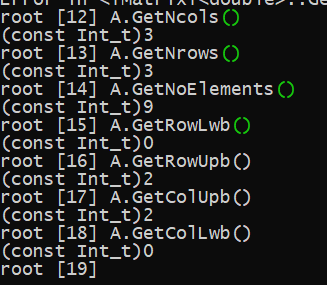I ping again @moneta who is our expert in that area.

Take your time, it’s ok. He might be busy working. Sorry for the long message.

`GetSub`” returns a submatrix `[row_lwb ... row_upb] x [col_lwb ... col_upb]`, so …
`A.GetSub(0,2,0,2)`” needs at least 3x3 matrix “`A`”.
`A.GetSub(3,5,3,5)`” needs at least 6x6 matrix “`A`”.
`A.GetSub(6,8,6,8)`” needs at least 9x9 matrix “`A`”.

1 Like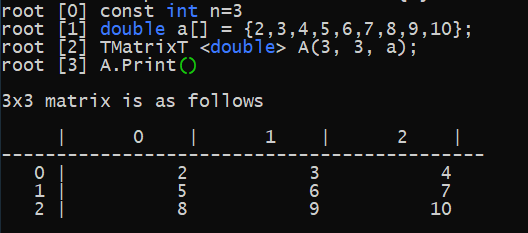Imagine we construct our 3x3 matrix as above. These 0, 1, 2 indexes on the top row and on the right column are referring to the same lower and upper bounds for rows and columns in our “GetSub (Int_t row_lwb, Int_t row_upb, Int_t col_lwb, Int_t col_upb, Option_t *option=“S”)” method. Is this correct?

In this 3x3 matrix, I can extract sub matrices whose biggest one will be itself, 3x3 matrix. When that’s the case, I wanna know what this part, I quaote below, is doing on a visualizable example.
`GetSub` ” returns a submatrix `[row_lwb ... row_upb] x [col_lwb ... col_upb]` , so …
`A.GetSub(0,2,0,2)` ” needs at least 3x3 matrix “`A` ”.
How can I Print() the submatrix you have just retrieved by A.GetSub(0,2,0,2)?

I have just found this link to investigate :https://root.cern.ch/root/html534/guides/users-guide/LinearAlgebra.html
I feel like so many similar pages on ROOT website about matrix to refer, so I find itconfusing. There are some typo error and some examples give error, but I will mention it later.

`GetSub`” returns just another matrix, so you can use any matrix method you want on it.

TMatrixT< Element > GetSub (Int_t row_lwb, Int_t row_upb, Int_t col_lwb, Int_t col_upb, Option_t *option=“S”) const

It’s obvious that it turns to a matrix, but I need to see an working example.

In this link, auto type gives an error.
Also, in the another link, there is this tiny bit of code, pasted below, related to my question. However, it is not really an example I need.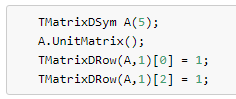`A.GetSub(0,2,0,2).Print();`

1 Like

A.GetSub(0,2,0,2).Print() ; this seems giving the same matrix as A. The matrix itself is one of the submatrices. Until that point, it made sense a bit.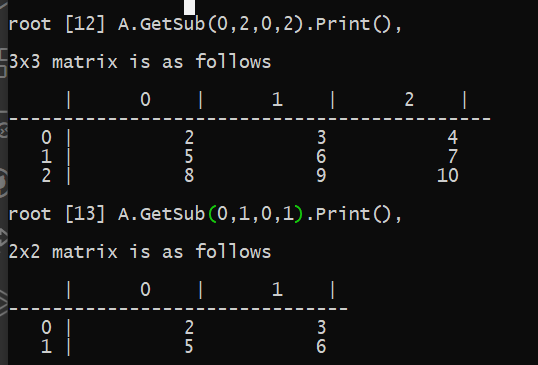As far as the picture concerns above, when we put 0 to 2 for row_Lwb and row_Upb, it gets first two rows. On the other hand, it gets first two columns when we put 0 to 2 for col_Lwb and col_Upb. This is actually what is does, doesn’t it! I got it!

It gives 3x3 matrix as you said. Let me play with that for a while. You haven’t given anyting about TMatrixDRow, … to access each element as an example yet!

@Wile_E_Coyote : Since, now, I understand the GetSub(…) method, can you tell me how the coverance matrix is created if you retrieve it from the Fit information as in this example above. Which bit from these 3 functions GetCoveranceMatrix() method is getting to create its matrix?

I seems to me, it has to be 9x9 matrix.Then, for instance, GetSub(0,2,0,2) part cuts and gets the matrix corresponding to the first two rows and cols of 9x9 covariance matrix.

See the “Access to the fit result” in the `TH1::Fit` method description.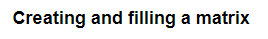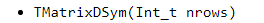@Wile_E_Coyote

From here, I understand that the Covmat is created from a row matrix of 9 parameters with TMatrixDSym. Covariance matrix should show the relation between the two, for instance X and Y, so a matrix with 9 rows will not be enough to get covmatrix.

Can you tell me now what will be our X matrix constituted from 2 Gaussian and 1 Pol2 functions, namely, 9 parameters in total to get cov_matrix (= (X^T .X) / Nrows )?

I want to make excel file myself like in that video, but I am not sure how to construct the X matrix in the first place?

The ("`npar`" x “`npar`”) covariance matrix is (always) a real symmetric (square) matrix, which (in general) has “`npar * (npar + 1) / 2`” independent elements (values).